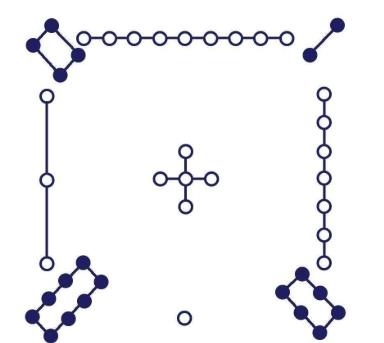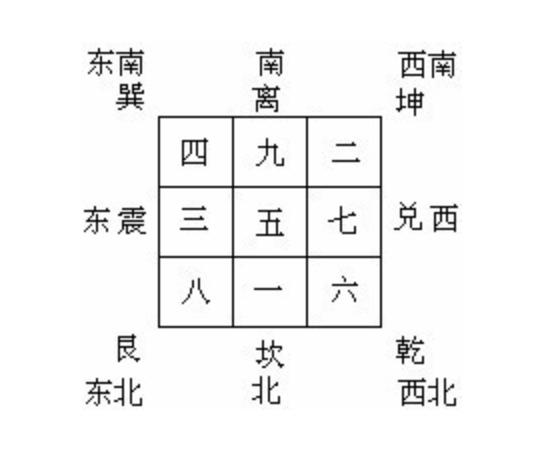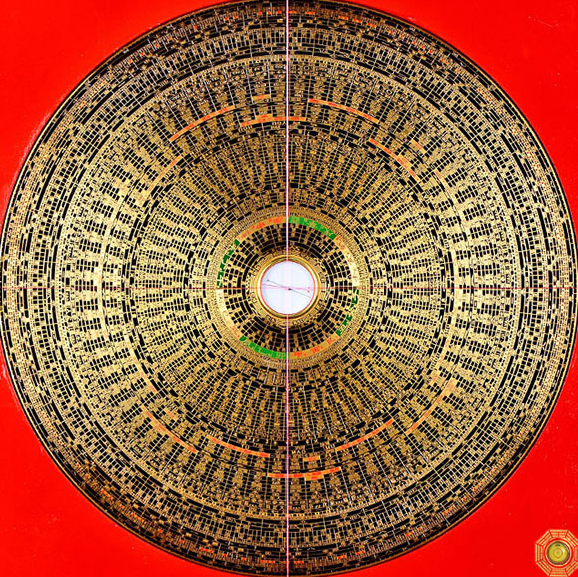# 道教大兴 万古大局

《黄帝内经-素问-上古天真论》：上古之人，其知道者，法于阴阳，和于术数。4    9    2

3    5    7

8    1    6

4+9+2=15

3+5+7=15

8+1+6=15

4+3+8=15

3+9+4 = 16     6

1+7+6 = 14     4

7+9+2 = 18     8

1+3+8 = 12     204，10，02
03，05，07
08，01，06

07，13，05
06，08，11
12，04，10

7 , 3 , 5

6 , 8,  1

2 , 4 , 9———-